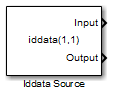# Iddata Source

Import time-domain data stored in `iddata` object in MATLAB workspace

• Library:
• System Identification Toolbox

•## Description

The Iddata Source block imports the input-output time-domain data stored in an `iddata` object in the MATLAB® workspace. You can use this block to import data for simulating a model in Simulink®.

## Ports

### Output

expand all

Input data stored in `iddata` object, returned as a scalar for single-input data and a vector of length Nu for multichannel data with Nu inputs. If `z` is the `iddata` object, the output at this port at simulation time t is `z.InputData` at time t. If t is greater than `z.SamplingInstants(end)`, the maximum time in `z`, the input data is returned as `0` for each input channel.

Data Types: `double`

Output data stored in `iddata` object, returned as a scalar for single-output data and as a vector of length Ny for multichannel data with Ny outputs. If `z` is the `iddata` object, the output at this port at simulation time t is `z.OutputData` at time t. If t is greater than `z.SamplingInstants(end)`, the maximum time in `z`, the output data is returned as `0` for each output channel.

Data Types: `double`

## Parameters

expand all

Time-domain data that is to be imported, specified as an `iddata` object that you have created in the MATLAB workspace. The `iddata` object must contain only one experiment. For a multiple-experiment `iddata` object `z`, to specify an `iddata` object for experiment number `kexp`, specify IDDATA object as `getexp(z,kexp)`.

## Extended Capabilities

### Topics

Introduced in R2008a

## SupportGet trial now